Next: The Rotating Reference Frame Up: Measuring the penetration depth with TF-SR Previous: Modelling The Asymmetry Spectrum of the Vortex State

# 4-Counter Geometry and the Complex Polarization

In the 2-counter setup of Fig. 3.11, information is lost by failing to detect decay positrons emitted in the up and down directions. A more efficient setup is the 4-counter arrangement depicted in Fig. 3.13, where additional counters are placed on the y and -y axes. The L and R counters monitor the x-component of the polarization Px(t) as before, while the U and D counters measure the y-component of the polarization Py(t). Ignoring geometric misalignments and differences in counter efficiency, Py(t) differs from Px(t) only by a phase of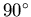. In terms of the field distribution n(B), Px(t) and Py(t)are defined as: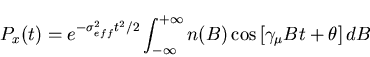(40)

 Py(t) =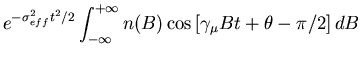=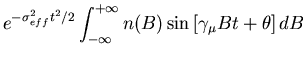(41)

where,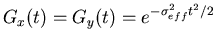. For an applied field in the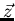-direction, the muon spin polarization transverse to the magnetic field Bz may be described by the complex quantity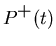=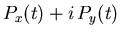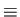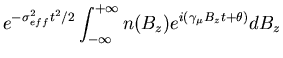(42)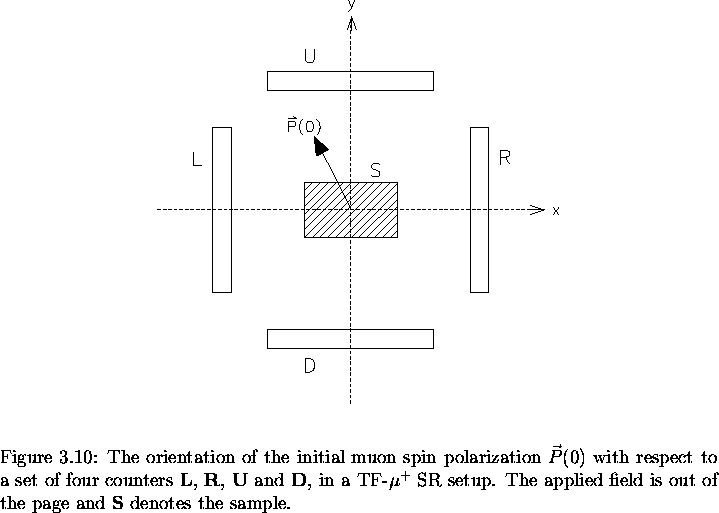The real Fourier transform of the complex muon polarization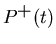approximates the field distribution n(Bz) with statistical noise due to finite counting rates . At the later times in the asymmetry spectrum, most of the muons have already decayed. With few muons left, the statistics at these later times are low, resulting in increased noise at the end of the asymmetry spectrum. Using the fact thatis defined only for positive times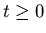, the field distribution n(Bz) may be written: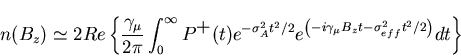(43)

where the gaussian apodization parameter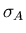is chosen to provide a compromise between statistical noise in the spectrum and additional broadening of the spectrum which such a procedure introduces.

The complex asymmetry for the 4-counter setup is defined as: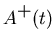=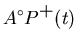=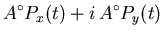=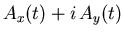(44)

where Ax(t) and Ay(t) are the real and imaginary parts of the complex asymmetry, respectively. The number of counts per second in the ith counter [i = R (right), L (left), U (up) or D (down)], may be written: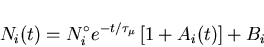(45)

where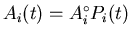is the asymmetry function for the ith raw histogram. Rearranging Eq. (3.45):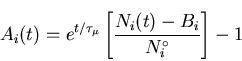(46)

In terms of the individual counters depicted in Fig. 3.13, the real asymmetry Ax(t) and the imaginary asymmetry Ay(t) may be written: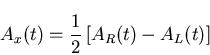(47)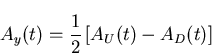(48)

In the present study, the real and imaginary parts of the asymmetry are fit simultaneously. For the vortex state of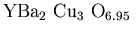, the real asymmetry can be fit with Eq. (3.39). The imaginary part of the asymmetry can be fit with the same function, but with a phase difference of.Next: The Rotating Reference Frame Up: Measuring the penetration depth with TF-SR Previous: Modelling The Asymmetry Spectrum of the Vortex State
Jess H. Brewer
2001-09-28